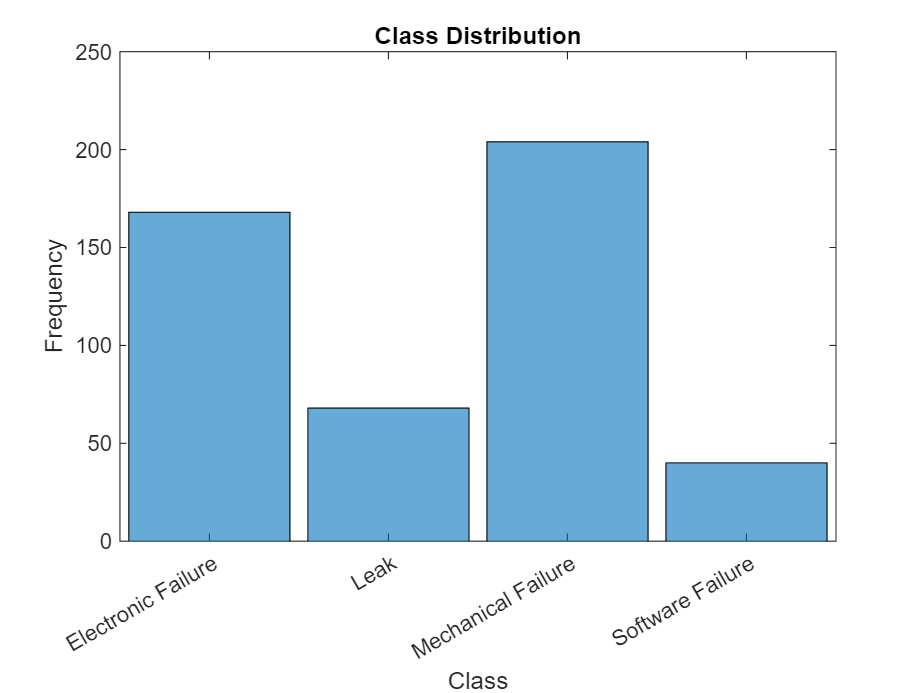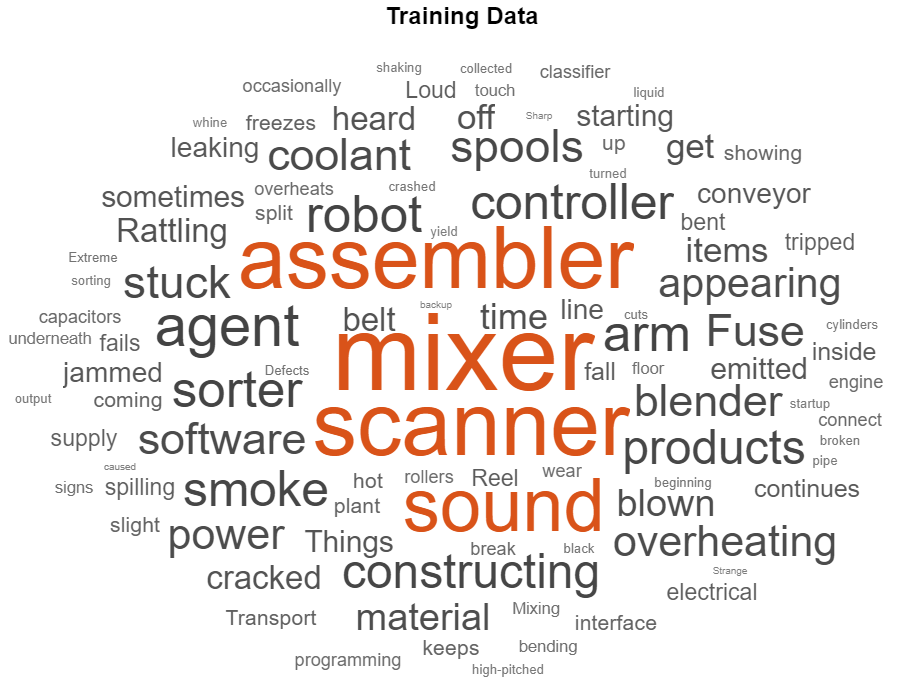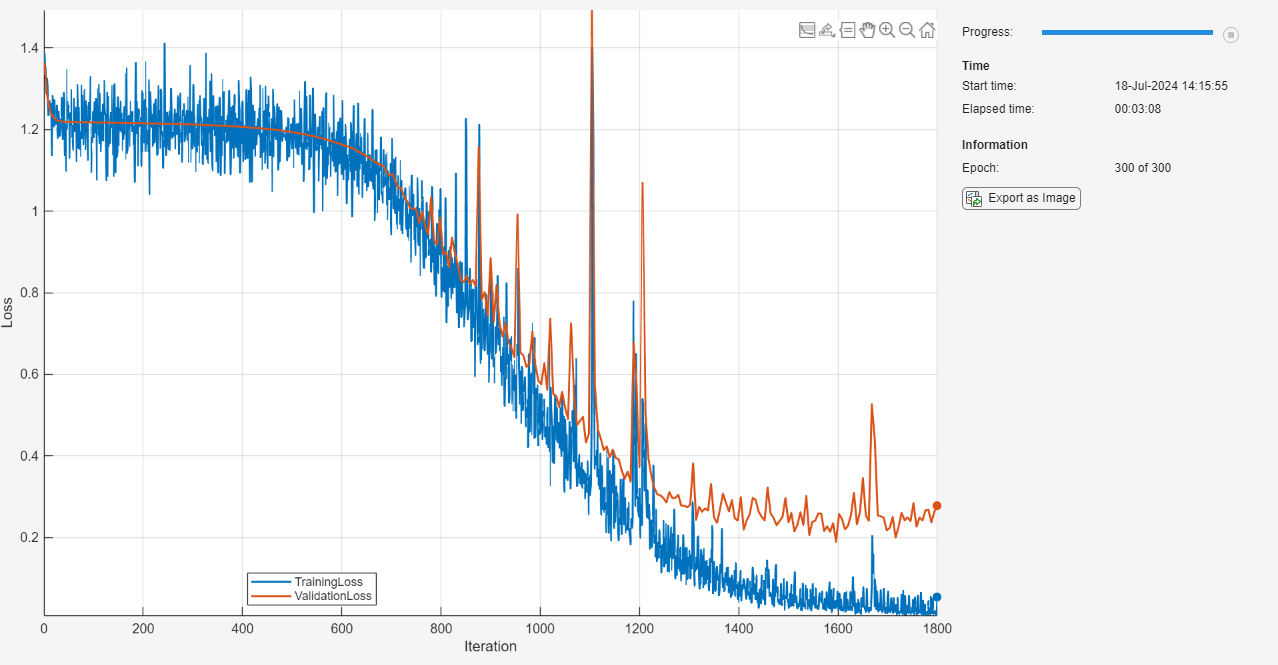# Classify Text Data Using Custom Training Loop

This example shows how to classify text data using a deep learning bidirectional long short-term memory (BiLSTM) network with a custom training loop.

When training a deep learning network using the `trainNetwork` function, if `trainingOptions` does not provide the options you need (for example, a custom learning rate schedule), then you can define your own custom training loop using automatic differentiation. For an example showing how to classify text data using the `trainNetwork` function, see Classify Text Data Using Deep Learning.

This example trains a network to classify text data with the time-based decay learning rate schedule: for each iteration, the solver uses the learning rate given by ${\rho }_{t}=\frac{{\rho }_{0}}{1+kt}$, where t is the iteration number, ${\rho }_{0}$ is the initial learning rate, and k is the decay.

### Import Data

Import the factory reports data. This data contains labeled textual descriptions of factory events. To import the text data as strings, specify the text type to be `"string"`.

```filename = "factoryReports.csv"; data = readtable(filename,TextType="string"); head(data)```
```ans=8×5 table Description Category Urgency Resolution Cost _____________________________________________________________________ ____________________ ________ ____________________ _____ "Items are occasionally getting stuck in the scanner spools." "Mechanical Failure" "Medium" "Readjust Machine" 45 "Loud rattling and banging sounds are coming from assembler pistons." "Mechanical Failure" "Medium" "Readjust Machine" 35 "There are cuts to the power when starting the plant." "Electronic Failure" "High" "Full Replacement" 16200 "Fried capacitors in the assembler." "Electronic Failure" "High" "Replace Components" 352 "Mixer tripped the fuses." "Electronic Failure" "Low" "Add to Watch List" 55 "Burst pipe in the constructing agent is spraying coolant." "Leak" "High" "Replace Components" 371 "A fuse is blown in the mixer." "Electronic Failure" "Low" "Replace Components" 441 "Things continue to tumble off of the belt." "Mechanical Failure" "Low" "Readjust Machine" 38 ```

The goal of this example is to classify events by the label in the `Category` column. To divide the data into classes, convert these labels to categorical.

`data.Category = categorical(data.Category);`

View the distribution of the classes in the data using a histogram.

```figure histogram(data.Category); xlabel("Class") ylabel("Frequency") title("Class Distribution")```The next step is to partition it into sets for training and validation. Partition the data into a training partition and a held-out partition for validation and testing. Specify the holdout percentage to be 20%.

```cvp = cvpartition(data.Category,Holdout=0.2); dataTrain = data(training(cvp),:); dataValidation = data(test(cvp),:);```

Extract the text data and labels from the partitioned tables.

```textDataTrain = dataTrain.Description; textDataValidation = dataValidation.Description; TTrain = dataTrain.Category; TValidation = dataValidation.Category;```

To check that you have imported the data correctly, visualize the training text data using a word cloud.

```figure wordcloud(textDataTrain); title("Training Data")```View the number of classes.

```classes = categories(TTrain); numClasses = numel(classes)```
```numClasses = 4 ```

### Preprocess Text Data

Create a function that tokenizes and preprocesses the text data. The function `preprocessText`, listed at the end of the example, performs these steps:

1. Tokenize the text using `tokenizedDocument`.

2. Convert the text to lowercase using `lower`.

3. Erase the punctuation using `erasePunctuation`.

Preprocess the training data and the validation data using the `preprocessText` function.

```documentsTrain = preprocessText(textDataTrain); documentsValidation = preprocessText(textDataValidation);```

View the first few preprocessed training documents.

`documentsTrain(1:5)`
```ans = 5×1 tokenizedDocument: 9 tokens: items are occasionally getting stuck in the scanner spools 10 tokens: loud rattling and banging sounds are coming from assembler pistons 5 tokens: fried capacitors in the assembler 4 tokens: mixer tripped the fuses 9 tokens: burst pipe in the constructing agent is spraying coolant ```

Create a single datastore that contains both the documents and the labels by creating `arrayDatastore` objects, then combining them using the `combine` function.

```dsDocumentsTrain = arrayDatastore(documentsTrain,OutputType="cell"); dsTTrain = arrayDatastore(TTrain,OutputType="cell"); dsTrain = combine(dsDocumentsTrain,dsTTrain);```

Create an array datastore for the validation documents.

`dsDocumentsValidation = arrayDatastore(documentsValidation,OutputType="cell");`

### Create Word Encoding

To input the documents into a BiLSTM network, use a word encoding to convert the documents into sequences of numeric indices.

To create a word encoding, use the `wordEncoding` function.

`enc = wordEncoding(documentsTrain)`
```enc = wordEncoding with properties: NumWords: 417 Vocabulary: ["items" "are" "occasionally" "getting" "stuck" "in" "the" "scanner" "spools" "loud" "rattling" "and" "banging" "sounds" "coming" "from" "assembler" "pistons" "fried" … ] ```

### Define Network

Define the BiLSTM network architecture. To input sequence data into the network, include a sequence input layer and set the input size to 1. Next, include a word embedding layer of dimension 25 and the same number of words as the word encoding. Next, include a BiLSTM layer and set the number of hidden units to 40. To use the BiLSTM layer for a sequence-to-label classification problem, set the output mode to `"last"`. Finally, add a fully connected layer with the same size as the number of classes, and a softmax layer.

```inputSize = 1; embeddingDimension = 25; numHiddenUnits = 40; numWords = enc.NumWords; layers = [ sequenceInputLayer(inputSize) wordEmbeddingLayer(embeddingDimension,numWords) bilstmLayer(numHiddenUnits,OutputMode="last") fullyConnectedLayer(numClasses) softmaxLayer]```
```layers = 5×1 Layer array with layers: 1 '' Sequence Input Sequence input with 1 dimensions 2 '' Word Embedding Layer Word embedding layer with 25 dimensions and 417 unique words 3 '' BiLSTM BiLSTM with 40 hidden units 4 '' Fully Connected 4 fully connected layer 5 '' Softmax softmax ```

Convert the layer array to a `dlnetwork` object.

`net = dlnetwork(layers)`
```net = dlnetwork with properties: Layers: [5×1 nnet.cnn.layer.Layer] Connections: [4×2 table] Learnables: [6×3 table] State: [2×3 table] InputNames: {'sequenceinput'} OutputNames: {'softmax'} Initialized: 1 ```

### Define Model Loss Function

Create the function `modelLoss`, listed at the end of the example, that takes a `dlnetwork` object, a mini-batch of input data with corresponding labels, and returns the loss and the gradients of the loss with respect to the learnable parameters in the network.

### Specify Training Options

Train for 30 epochs with a mini-batch size of 16.

```numEpochs = 30; miniBatchSize = 16;```

Specify the options for Adam optimization. Specify an initial learn rate of 0.001 with a decay of 0.01, gradient decay factor 0.9, and squared gradient decay factor 0.999.

```initialLearnRate = 0.001; decay = 0.01; gradientDecayFactor = 0.9; squaredGradientDecayFactor = 0.999;```

### Train Model

Create a `minibatchqueue` object that processes and manages the mini-batches of data. For each mini-batch:

• Use the custom mini-batch preprocessing function `preprocessMiniBatch` (defined at the end of this example) to convert documents to sequences and one-hot encode the labels. To pass the word encoding to the mini-batch, create an anonymous function that takes two inputs.

• Format the predictors with the dimension labels `"BTC"` (batch, time, channel). The `minibatchqueue` object, by default, converts the data to `dlarray` objects with underlying type `single`.

• Train on a GPU if one is available. The `minibatchqueue` object, by default, converts each output to `gpuArray` if a GPU is available. Using a GPU requires Parallel Computing Toolbox™ and a supported GPU device. For information on supported devices, see GPU Support by Release (Parallel Computing Toolbox).

```mbq = minibatchqueue(dsTrain, ... MiniBatchSize=miniBatchSize,... MiniBatchFcn=@(X,T) preprocessMiniBatch(X,T,enc), ... MiniBatchFormat=["BTC" ""]);```

Create a `minibatchqueue` object for the validation documents. For each mini-batch:

• Use the custom mini-batch preprocessing function `preprocessMiniBatchPredictors` (defined at the end of this example) to convert documents to sequences. This preprocessing function does not require label data. To pass the word encoding to the mini-batch, create an anonymous function that takes one input only.

• Format the predictors with the dimension labels `"BTC"` (batch, time, channel). The `minibatchqueue` object, by default, converts the data to `dlarray` objects with underlying type `single`.

• To make predictions for all observations, return any partial mini-batches.

```mbqValidation = minibatchqueue(dsDocumentsValidation, ... MiniBatchSize=miniBatchSize, ... MiniBatchFcn=@(X) preprocessMiniBatchPredictors(X,enc), ... MiniBatchFormat="BTC", ... PartialMiniBatch="return");```

To easily calculate the validation loss, convert the validation labels to one-hot encoded vectors and transpose the encoded labels to match the network output format.

```TValidation = onehotencode(TValidation,2); TValidation = TValidation';```

Initialize the training progress plot.

```figure C = colororder; lineLossTrain = animatedline(Color=C(2,:)); lineLossValidation = animatedline( ... LineStyle="--", ... Marker="o", ... MarkerFaceColor="black"); ylim([0 inf]) xlabel("Iteration") ylabel("Loss") grid on```

```trailingAvg = []; trailingAvgSq = [];```

Train the network. For each epoch, shuffle the data and loop over mini-batches of data. At the end of each iteration, display the training progress. At the end of each epoch, validate the network using the validation data.

For each mini-batch:

• Convert the documents to sequences of integers and one-hot encode the labels.

• Convert the data to `dlarray` objects with underlying type single and specify the dimension labels `"BTC"` (batch, time, channel).

• For GPU training, convert to `gpuArray` objects.

• Evaluate the model loss and gradients using `dlfeval` and the `modelLoss` function.

• Determine the learning rate for the time-based decay learning rate schedule.

• Update the network parameters using the `adamupdate` function.

• Update the training plot.

```iteration = 0; start = tic; % Loop over epochs. for epoch = 1:numEpochs % Shuffle data. shuffle(mbq); % Loop over mini-batches. while hasdata(mbq) iteration = iteration + 1; % Read mini-batch of data. [X,T] = next(mbq); % Evaluate the model loss and gradients using dlfeval and the % modelLoss function. [loss,gradients] = dlfeval(@modelLoss,net,X,T); % Determine learning rate for time-based decay learning rate schedule. learnRate = initialLearnRate/(1 + decay*iteration); % Update the network parameters using the Adam optimizer. [net,trailingAvg,trailingAvgSq] = adamupdate(net, gradients, ... trailingAvg, trailingAvgSq, iteration, learnRate, ... gradientDecayFactor, squaredGradientDecayFactor); % Display the training progress. D = duration(0,0,toc(start),Format="hh:mm:ss"); loss = double(loss); addpoints(lineLossTrain,iteration,loss) title("Epoch: " + epoch + ", Elapsed: " + string(D)) drawnow % Validate network. if iteration == 1 || ~hasdata(mbq) [~,scoresValidation] = modelPredictions(net,mbqValidation,classes); lossValidation = crossentropy(scoresValidation,TValidation); % Update plot. lossValidation = double(lossValidation); addpoints(lineLossValidation,iteration,lossValidation) drawnow end end end```### Test Model

Test the classification accuracy of the model by comparing the predictions on the validation set with the true labels.

Classify the validation data using `modelPredictions` function, listed at the end of the example.

`YNew = modelPredictions(net,mbqValidation,classes);`

To easily calculate the validation accuracy, convert the one-hot encoded validation labels to categorical and transpose.

`TValidation = onehotdecode(TValidation,classes,1)';`

Evaluate the classification accuracy.

`accuracy = mean(YNew == TValidation)`
```accuracy = 0.8854 ```

### Predict Using New Data

Classify the event type of three new reports. Create a string array containing the new reports.

```reportsNew = [ "Coolant is pooling underneath sorter." "Sorter blows fuses at start up." "There are some very loud rattling sounds coming from the assembler."];```

Preprocess the text data using the preprocessing steps as the training documents.

```documentsNew = preprocessText(reportsNew); dsNew = arrayDatastore(documentsNew,OutputType="cell");```

Create a `minibatchqueue` object that processes and manages the mini-batches of data. For each mini-batch:

• Use the custom mini-batch preprocessing function `preprocessMiniBatchPredictors` (defined at the end of this example) to convert documents to sequences. This preprocessing function does not require label data. To pass the word encoding to the mini-batch, create an anonymous function that takes one input only.

• Format the predictors with the dimension labels `"BTC"` (batch, time, channel). The `minibatchqueue` object, by default, converts the data to `dlarray` objects with underlying type `single`.

• To make predictions for all observations, return any partial mini-batches.

```mbqNew = minibatchqueue(dsNew, ... MiniBatchSize=miniBatchSize, ... MiniBatchFcn=@(X) preprocessMiniBatchPredictors(X,enc), ... MiniBatchFormat="BTC", ... PartialMiniBatch="return");```

Classify the text data using `modelPredictions` function, listed at the end of the example and find the classes with the highest scores.

`YNew = modelPredictions(net,mbqNew,classes)`
```YNew = 3×1 categorical Leak Electronic Failure Mechanical Failure ```

### Supporting Functions

#### Text Preprocessing Function

The function `preprocessText` performs these steps:

1. Tokenize the text using `tokenizedDocument`.

2. Convert the text to lowercase using `lower`.

3. Erase the punctuation using `erasePunctuation`.

```function documents = preprocessText(textData) % Tokenize the text. documents = tokenizedDocument(textData); % Convert to lowercase. documents = lower(documents); % Erase punctuation. documents = erasePunctuation(documents); end```

#### Mini-Batch Preprocessing Function

The `preprocessMiniBatch` function converts a mini-batch of documents to sequences of integers and one-hot encodes label data.

```function [X,T] = preprocessMiniBatch(dataX,dataT,enc) % Preprocess predictors. X = preprocessMiniBatchPredictors(dataX,enc); % Extract labels from cell and concatenate. T = cat(1,dataT{1:end}); % One-hot encode labels. T = onehotencode(T,2); % Transpose the encoded labels to match the network output. T = T'; end```

#### Mini-Batch Predictors Preprocessing Function

The `preprocessMiniBatchPredictors` function converts a mini-batch of documents to sequences of integers.

```function X = preprocessMiniBatchPredictors(dataX,enc) % Extract documents from cell and concatenate. documents = cat(4,dataX{1:end}); % Convert documents to sequences of integers. X = doc2sequence(enc,documents); X = cat(1,X{:}); end```

#### Model Loss Function

The `modelLoss` function takes a `dlnetwork` object `net`, a mini-batch of input data `X` with corresponding target labels `T` and returns the gradients of the loss with respect to the learnable parameters in `net`, and the loss. To compute the gradients automatically, use the `dlgradient` function.

```function [loss,gradients] = modelLoss(net,X,T) Y = forward(net,X); loss = crossentropy(Y,T); gradients = dlgradient(loss,net.Learnables); end```

#### Model Predictions Function

The `modelPredictions` function takes a `dlnetwork` object `net`, a mini-batch queue, and outputs the model predictions and scores by iterating over mini-batches in the queue.

```function [predictions,scores] = modelPredictions(net,mbq,classes) % Initialize predictions. predictions = []; scores = []; % Reset mini-batch queue. reset(mbq); % Loop over mini-batches. while hasdata(mbq) % Make predictions. X = next(mbq); Y = predict(net,X); scores = [scores Y]; Y = onehotdecode(Y,classes,1)'; predictions = [predictions; Y]; end end```